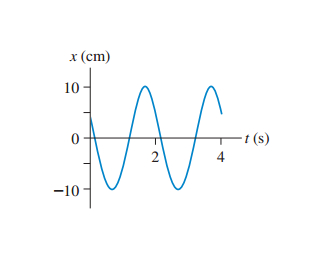# Problem: (Figure 1) shows a position-versus-time graph for a particle in SHM.A) What is the amplitude A?B) What is the angular frequency ω?C) What is the phase constant ϕ0?

###### FREE Expert Solution

The position versus time equation is:

$\overline{){\mathbf{x}}{\mathbf{\left(}}{\mathbf{t}}{\mathbf{\right)}}{\mathbf{=}}{\mathbf{A}}{\mathbf{c}}{\mathbf{o}}{\mathbf{s}}{\mathbf{\left(}}{\mathbf{\omega }}{\mathbf{t}}{\mathbf{+}}{{\mathbf{\varphi }}}_{{\mathbf{0}}}{\mathbf{\right)}}}$

The angular frequency:

$\overline{){\mathbf{\omega }}{\mathbf{=}}\frac{\mathbf{2}\mathbf{\pi }}{\mathbf{T}}}$

A)

Amplitude is the maximum change in the wave's position.

90% (271 ratings)###### Problem Details

(Figure 1) shows a position-versus-time graph for a particle in SHM.A) What is the amplitude A?

B) What is the angular frequency ω?

C) What is the phase constant ϕ0?